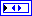Draw Mode Property

FlexRIO Help

Edition Date: November 2015

Part Number: 372614J-01

»View Product Info

Short Name: DrawMode

Requires: Full Development System

Class: SceneMesh Properties

To Use: Create a property.

Returns the mode used to draw the mesh.

Values

 0 Points—LabVIEW draws a point at each of the n vertices. 1 Lines—LabVIEW draws the mesh as a series of unconnected line segments. A line connects vertices 0 and 1, a second line connects vertices 2 and 3, and so on. 2 Line Strip—LabVIEW draws the mesh as a series of connected lines. The line between vertices 0 and 1 connects to the line between vertices 1 and 2, and so on. 3 Line Loop—Similar to Line Strip, LabVIEW draws the mesh as a series of connected lines, but connects the final vertex back to the original vertex, completing a loop. 4 Triangles—LabVIEW draws the mesh using a series of triangles with points defined by groups of three vertices. The first triangle connects vertices 0, 1, and 2, the second triangle connects vertices 3, 4, and 5, and so on. If the total number of vertices in the mesh is not a multiple of 3, LabVIEW ignores the final one or two vertices. 5 Triangle Strip—LabVIEW draws the mesh using a series of conjoined triangles that form part of a surface. The first triangle connects vertices 0, 1, and 2, the second triangle connects vertices 2, 1, and 3, and so on. The ordering preserves the orientation of the triangles. 6 Triangle Fan—Similar to Triangle Strip, LabVIEW draws the mesh using a series of conjoined triangles, but with a different ordering of the mesh's vertices. The first triangle connects vertices 0, 1, and 2, the second triangle connects vertices 0, 2, and 3, and so on to create a fan shape. 7 Quads—LabVIEW draws the mesh using a series of quadrilaterals. The first quad connects vertices 0, 1, 2, and 3, the second quad connects vertices 4, 5, 6, and 7, and so on. If the total number of vertices is not a multiple of 4, LabVIEW ignores the final one, two, or three vertices. 8 Quad Strip—LabVIEW draws the mesh using a series of conjoined quadrilaterals. The first quad connects vertices 0, 1, 3, and 2, the second quad connects vertices 2, 3, 5, and 4, and so on. If the total number of vertices is odd, LabVIEW ignores the final vertex. 9 Polygon—LabVIEW draws the mesh as a polygon, connecting all vertices in the mesh and filling the space between them. To use this draw mode, the polygon must be convex and must not intersect itself at any point.

Remarks

The following table lists the characteristics of this property.

 Data typePermissions Read Only Available in Run-Time Engine Yes Available in Real-Time Operating System No Settable when the VI is running No Loads the front panel into memory No Need to authenticate before use No Loads the block diagram into memory No Remote access allowed Yes